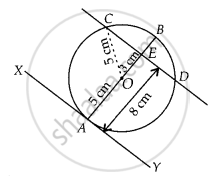# At one end A of a diameter AB of a circle of radius 5 cm, tangent XAY is drawn to the circle. The length of the chord CD parallel to XY and at a distance of 8 cm from A is ______ - Mathematics

MCQ
Fill in the Blanks

At one end A of a diameter AB of a circle of radius 5 cm, tangent XAY is drawn to the circle. The length of the chord CD parallel to XY and at a distance of 8 cm from A is ______

• 4 cm

• 5 cm

• 6 cm

• 8 cm

#### Solution

At one end A of a diameter AB of a circle of radius 5 cm, tangent XAY is drawn to the circle. The length of the chord CD parallel to XY and at a distance of 8 cm from A is 8 cm.

Explanation:

First, draw a circle of radius 5 cm with centre O. A tangent XY is drawn at point A.A chord CD is drawn which is parallel to XY and at a distance of 8 cm from A.

Now, ∠OAY = 90°  ......[∵ Tangent at any point of circle is perpendicular to the radius through the point of contact]

∠OAY +∠OED= 180°   .......[∵ Sum of cointerior angles is 180°]

⇒ ∠OED = 180° – 90° = 90°

Also, AE = 8 cm Join OC.

OC = 5 cm  ......[Radius of circle]

OE = AE – OA = 8 – 5 = 3 cm

Now, in right angled ∆OEC,

OC2 = OE2 + EC2   ......[By Pythagoras theorem]

⇒ EC2 = OC2 – OE2

⇒ EC2 = 52 – 32

⇒ EC2 = 25 – 9 = 16

⇒ EC = 4 cm

Since, perpendicular from centre to the chord bisects the chord.

∴ CE = ED

⇒ CD = 2 × EC

⇒ CD = 2 × 4

⇒ CD = 8 cm

Concept: Number of Tangents from a Point on a Circle
Is there an error in this question or solution?

#### APPEARS IN

NCERT Mathematics Exemplar Class 10
Chapter 9 Circles
Exercise 9.1 | Q 5 | Page 103
Share